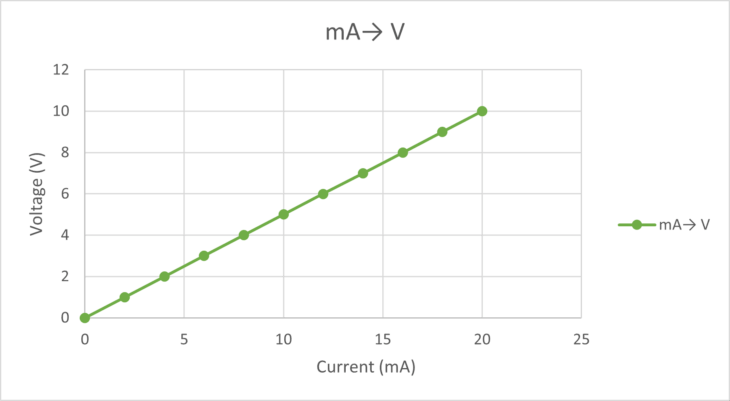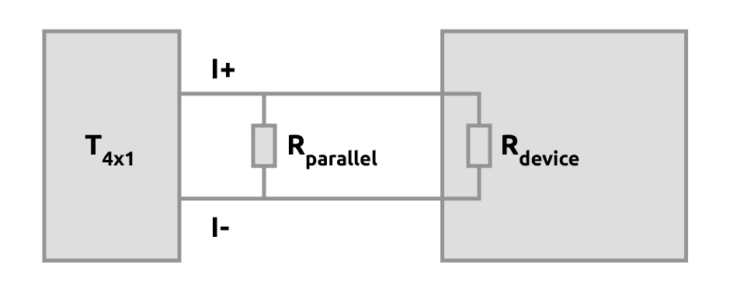# Application note: Jaquet T4x2 obsolescence and alternative

TE Connectivity has announced on 4th June 2021 to discontinue all special versions of the Jaquet T400 family. Only the T401 (383Z-05307) and T411 (384Z-05601) will still be commercially available. This means, that among others, the T402 and T412 speed transmitters are obsolete. In this application note we describe how the specific feature of T402 and T412, a 0(2)..10 V output signal, can be recreated with a T401 / T411.

## T402 / T412 alternative solution

There is a solution to recreate the 0(2)..10 V output. This can be achieved by using a T401 / T411 with a 500 Ω / 0.1% / ¼ W resistor parallel to its (0)4-20 mA output.

In the figures below the connection details are shown. The above mentioned resistor needs to be placed parallel with the -I and +I terminals.Figure 1: Jaquet T411 with a 500 Ω / 0.1% / ¼ W resistor parallel to its (0)4-20 mA outputFigure 2: Jaquet T401 with a 500 Ω / 0.1% / ¼ W resistor parallel to its (0)4-20 mA output

The (0)2..10 V output voltage is then almost directly proportional to the T401’s (0)4..20 mA output signal as shown in figure 3.Figure 3: Relation between (0)4..20mAmp out and (0)2-10 Volt conversion using a parallel 500 Ω resistor.

## Considerations and examples

Note that it is required to verify that the input impedance of the measuring system is ≥ 100 kΩ to minimize the error.

We can see this by comparing two calculation examples with 10 kΩ and 100 kΩ as the device’s impedance.Figure 4: Rparallel = 500 Ω, Rdevice = input impedance of measuring system.

Example 1: when Rdevice = 10 kΩ, the total resistance will be 476 Ω. @ 20 mA the voltage output will then be 9.52 Volt (error approx. 5%)

Example 2: when Rdevive = 100 kΩ, the total resistance will be 497 Ω. @ 20 mA the voltage output will then be 9.94 V (error approx. 0.6%)

It is clear that we want the 500 Ω parallel resistor to dominate the total resistance in the loop. This is achieved when the input impedance of the measuring system is ≥ 100 kΩ.